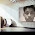## GRAVITATIONcredits: Dennis Nilson

MCQs

Q1: An object moving in (upward) direction opposite to the gravitational force of earth performs _________

(a) accelerated motion
(b) motion with constant velocity
(c) oscillations
(d) retarded motion

Q2: The value of acceleration due to gravity of the surface of the earth is

(a) 2 m/s2
(b) 4.9 m/s2
(c) 9.8 m/s2
(d) 8 m/s2

Q3: The mass of an object __________

(a) varies at different locations
(b) remains constant
(c) can be measured using spring balance
(d) is in the direction of gravitational force.

Q4: The value of acceleration due to gravity at the poles

(a) Is more than at the equator
(b) Same as at the equator
(c) Is less than at the equator
(d) zero

Q5: When an object is released freely from certain height without applying any external force, then the object

(a) will move upwards with accelerated motion
(b) will move downwards with accelerated motion
(c) will move downwards with constant velocity
(d) will move upwards with constant velocity

Q6: The gravitational force between two bodies is

(a) always repulsive
(b) always attractive
(c) can be attactive or repulsive
(d) neutral

Q7: Weight of an object on the surface of the moon is

(a) 1/5 th that on the surface of the earth
(b) 1/3 that on the surface of the earth
(c) ½ that on the surface of the earth
(d) 1/6 that on the surface of the earth

Q8: The force of attraction between two bodies (each having mass of 1kg) are kept at a distance of 1m w.r.t. each other is:

(a) 9.8 N
(b) 6.7 N
(c) 980 N
(d) 6.7 x 10 -11 N

Q9: The time of ascent when measured from the point of projection of a body projected upwards, the

(a) Time of ascent > Time of descent
(b) Time of ascent = Time of descent
(c) Time of ascent < Time of descent
(d) All of these

Q10: The relative density ___________

(a) has unit of kg/m3
(b) has unit of g/cm3
(c) has unit of kg/m2
(d) is unitless

How Newton discovered Laws Of Gravitation Watch Video Tutorial by NCERT Channel

1: (d) retarded motion
2: (c) 9.8 m/s2
3: (b) remains constant
4: (a) Is more than at the equator
5: (b) will move downwards with accelerated motion
6: (b) always attractive
7: (d) 1/6 that on the surface of the earth
8: (d) 6.7 x 10 -11 N
9: (b) Time of ascent = Time of descent
10:(d) is unitless1.nice.....very useful

2.Tanzzxxx ...its nice

3.This comment has been removed by the author.

4.THANKSSSSSSS

5.Nice , vry useful

6.Vry nice , useful , thankssssssss .